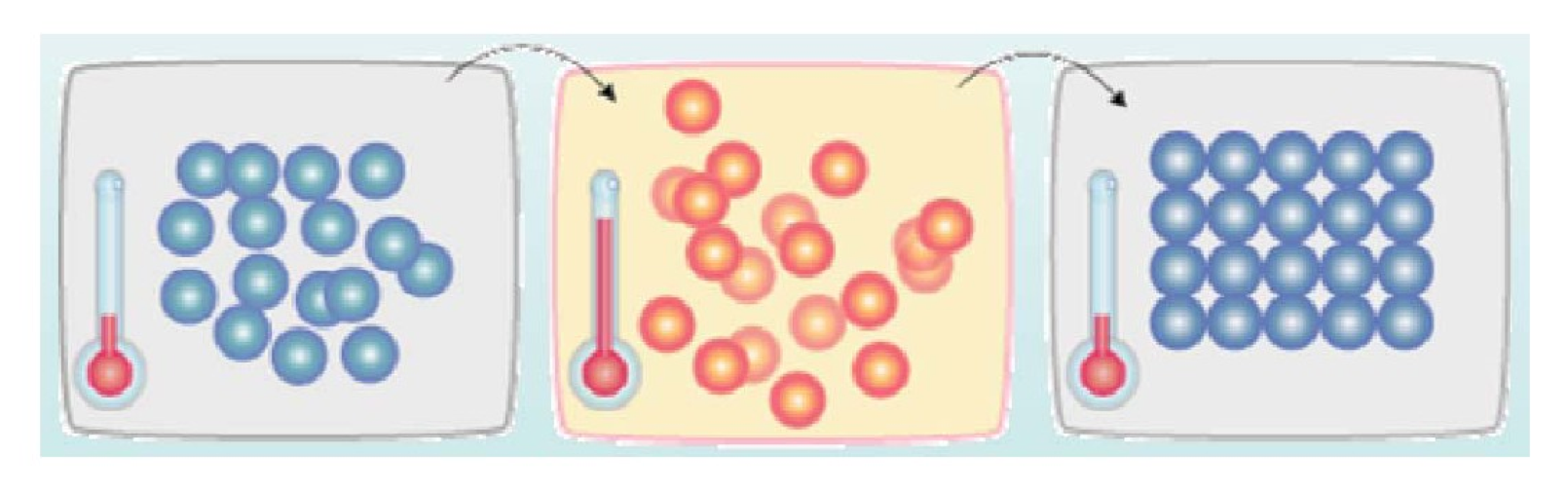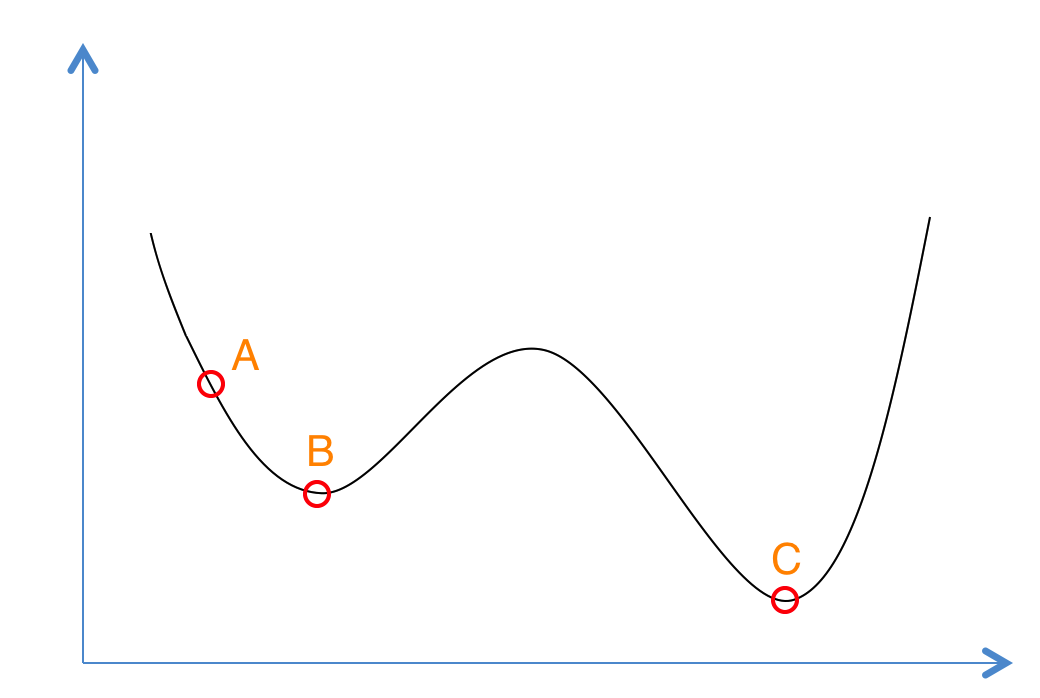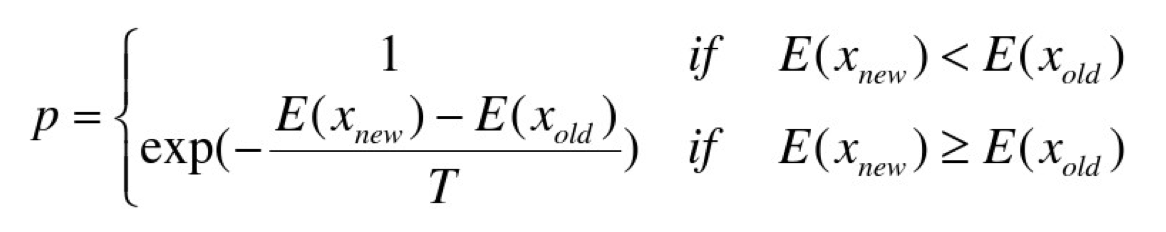模拟退火算法

著名的模拟退火算法，它是一种基于蒙特卡洛思想设计的近似求解最优化问题的方法。

美国物理学家 N.Metropolis 和同仁在1953年发表研究复杂系统、计算其中能量分布的文章，他们使用蒙特卡罗模拟法计算多分子系统中分子的能量分布。这相当于是本文所探讨之问题的开始，事实上，模拟退火中常常被提到的一个名词就是Metropolis准则，后面我们还会介绍。

Kirkpatrick等人受到Metropolis等人用蒙特卡罗模拟的启发而发明了“模拟退火”这个名词，因为它和物体退火过程相类似。寻找问题的最优解（最值）即类似寻找系统的最低能量。因此系统降温时，能量也逐渐下降，而同样意义地，问题的解也“下降”到最值。Metropolis准则表明，在温度为T时，出现能量差为dE的降温的概率为P(dE)，表示为：P(dE) = exp( dE/(kT) )。其中k是一个常数，exp表示自然指数，且dE<0。所以P和T正相关。这条公式就表示：温度越高，出现一次能量差为dE的降温的概率就越大；温度越低，则出现降温的概率就越小。又由于dE总是小于0（因为退火的过程是温度逐渐下降的过程），因此dE/kT < 0 ，所以P(dE)的函数取值范围是(0,1) 。随着温度T的降低，P(dE)会逐渐降低。

• f( Y(i+1) ) <= f( Y(i) )  (即移动后得到更优解)，则总是接受该移动；
• f( Y(i+1) ) > f( Y(i) )  (即移动后的解比当前解要差)，则以一定的概率接受移动，而且这个概率随着时间推移逐渐降低（逐渐降低才能趋向稳定）相当于上图中，从B移向BC之间的小波峰时，每次右移（即接受一个更糟糕值）的概率在逐渐降低。如果这个坡特别长，那么很有可能最终我们并不会翻过这个坡。如果它不太长，这很有可能会翻过它，这取决于衰减 t 值的设定。

• 普通Greedy算法：兔子朝着比现在低的地方跳去。它找到了不远处的最低的山谷。但是这座山谷不一定最低的。这就是普通Greedy算法，它不能保证局部最优值就是全局最优值。
• 模拟退火：兔子喝醉了。它随机地跳了很长时间。这期间，它可能走向低处，也可能踏入平地。但是，它渐渐清醒了并朝最低的方向跳去。这就是模拟退火。

通过一个实例来编程演示模拟退火的执行。特别地，我们这里所采用的实例是著名的“旅行商问题”（TSP，Traveling Salesman Problem），它是哈密尔顿回路的一个实例化问题，也是最早被提出的NP问题之一。

TSP是一个最常被用来解释模拟退火用法的问题，因为这个问题比较有名，我们这里不赘言重述，下面直接给出C++实现的代码：

#include <iostream>
#include <string.h>
#include <stdlib.h>
#include <algorithm>
#include <stdio.h>
#include <time.h>
#include <math.h>

#define N     30      //城市数量
#define T     3000    //初始温度
#define EPS   1e-8    //终止温度
#define DELTA 0.98    //温度衰减率

#define LIMIT 1000   //概率选择上限
#define OLOOP 20    //外循环次数
#define ILOOP 100   //内循环次数

using namespace std;

//定义路线结构体
struct Path
{
int citys[N];
double len;
};

//定义城市点坐标
struct Point
{
double x, y;
};

Path bestPath;        //记录最优路径
Point p[N];       //每个城市的坐标
double w[N][N];   //两两城市之间路径长度
int nCase;        //测试次数

double dist(Point A, Point B)
{
return sqrt((A.x - B.x) * (A.x - B.x) + (A.y - B.y) * (A.y - B.y));
}

void GetDist(Point p[], int n)
{
for(int i = 0; i < n; i++)
for(int j = i + 1; j < n; j++)
w[i][j] = w[j][i] = dist(p[i], p[j]);
}

void Input(Point p[], int &n)
{
scanf("%d", &n);
for(int i = 0; i < n; i++)
scanf("%lf %lf", &p[i].x, &p[i].y);
}

void Init(int n)
{
nCase = 0;
bestPath.len = 0;
for(int i = 0; i < n; i++)
{
bestPath.citys[i] = i;
if(i != n - 1)
{
printf("%d--->", i);
bestPath.len += w[i][i + 1];
}
else
printf("%d\n", i);
}
printf("\nInit path length is : %.3lf\n", bestPath.len);
printf("-----------------------------------\n\n");
}

void Print(Path t, int n)
{
printf("Path is : ");
for(int i = 0; i < n; i++)
{
if(i != n - 1)
printf("%d-->", t.citys[i]);
else
printf("%d\n", t.citys[i]);
}
printf("\nThe path length is : %.3lf\n", t.len);
printf("-----------------------------------\n\n");
}

Path GetNext(Path p, int n)
{
Path ans = p;
int x = (int)(n * (rand() / (RAND_MAX + 1.0)));
int y = (int)(n * (rand() / (RAND_MAX + 1.0)));
while(x == y)
{
x = (int)(n * (rand() / (RAND_MAX + 1.0)));
y = (int)(n * (rand() / (RAND_MAX + 1.0)));
}
swap(ans.citys[x], ans.citys[y]);
ans.len = 0;
for(int i = 0; i < n - 1; i++)
ans.len += w[ans.citys[i]][ans.citys[i + 1]];
cout << "nCase = " << nCase << endl;
Print(ans, n);
nCase++;
return ans;
}

void SA(int n)
{
double t = T;
srand((unsigned)(time(NULL)));
Path curPath = bestPath;
Path newPath = bestPath;
int P_L = 0;
int P_F = 0;
while(1)       //外循环，主要更新参数t，模拟退火过程
{
for(int i = 0; i < ILOOP; i++)    //内循环，寻找在一定温度下的最优值
{
newPath = GetNext(curPath, n);
double dE = newPath.len - curPath.len;
if(dE < 0)   //如果找到更优值，直接更新
{
curPath = newPath;
P_L = 0;
P_F = 0;
}
else
{
double rd = rand() / (RAND_MAX + 1.0);
//如果找到比当前更差的解，以一定概率接受该解，并且这个概率会越来越小
if(exp(dE / t) > rd && exp(dE / t) < 1)
curPath = newPath;
P_L++;
}
if(P_L > LIMIT)
{
P_F++;
break;
}
}
if(curPath.len < bestPath.len)
bestPath = curPath;
if(P_F > OLOOP || t < EPS)
break;
t *= DELTA;
}
}

int main(int argc, const char * argv[]) {

freopen("TSP.data", "r", stdin);
int n;
Input(p, n);
GetDist(p, n);
Init(n);
SA(n);
Print(bestPath, n);
printf("Total test times is : %d\n", nCase);
return 0;
}

public class Tour{
... ...
// Creates a random individual
public void generateIndividual() {
// Loop through all our destination cities and add them to our tour
for (int cityIndex = 0; cityIndex < TourManager.numberOfCities(); cityIndex++) {
setCity(cityIndex, TourManager.getCity(cityIndex));
}
// Randomly reorder the tour
Collections.shuffle(tour);
}
... ...
}

可见作者的方法是把上一轮路径做一个随机的重排（这显然也是一种策略）。

TSP.data的数据格式如下，第一行的数字表示一个有多少座城市，第2至最后一行，每行有两个数字表示，城市的坐标（平面直角坐标系）。例如：

20 80
16 84
23 66
62 90
11 9
35 28

参考文献与推荐阅读材料

【1】关于哈密尔顿问题和TSP问题请参考下面两个资料以了解更多：

【2】上面的C++代码在下面这个两个帖子中都有给出，原作者无法考证

【3】关于TSP问题的一个Java语言实现的源码，请参考

C/C++基本语法学习 STL C++ primer
posted @ 2016-11-20 23:54  ranjiewen  阅读(76765)  评论(7编辑  收藏  举报